# MP Board Class 11th Chemistry Important Questions Chapter 7 Equilibrium

## MP Board Class 11th Chemistry Important Questions Chapter 7 Equilibrium

### Equilibrium Important Questions

Equilibrium Objective Type Questions

Question 1.

Question 1.
Which of the following reaction have equal Kc and Kp:
(a) N2(g) + 3H2(g) ⇄ 2NH3
(b) 2H2S(g) + 3O2(g) ⇄ 2SO2(g) + 2H2O(g)
(c) Br2 + Cl2(g) ⇄ 2BrCl(g)
(d) P4(g) + 6Cl2(g) ⇄ 4PCl3(g)
(c) Br2 + Cl2(g) ⇄ 2BrCl(g)

Question 2.
For the reaction N2(g) + 3H2(g) ⇄ 2NH3(g), ∆H = 92 kJ, the concentration of NH3 at the equilibrium increase by temperature:
(a) Increase
(b) No change
(c) Decrease
(d) None of the above
(c) Decrease

Question 3.
In one litre container equilibrium mixture of reaction 2H2S(g) ⇄ 2H2S(g) + S2S(g) is filled 6.5 mol H2S. 0.1 mol H2 and 0.4 mol S2 are present in it. Equilibrium constant of this reaction will be:
(a) 0.004 mol litre-1
(b) 0.080 mol litre-1
(c) 0.016 mol litre-1
(d) 0.160 mol litre-1
(c) 0.016 mol litre-1Question 4.
Favourable condition for exothermic reaction of ammonia synthesis N2S(g) + 3H2S(g) ⇄ 2NH3(g) are:
(a) High temperature and high pressure
(b) High temperature and low pressure
(c) Low temperature and high pressure
(d) Low temperature and pressure
(c) Low temperature and high pressure

Question 5.
Oxidation of SO2 by O2 in SO3 is an exothermic reaction manufacture of SO3 will be maximum if:
(a) Temperature increased and pressure decreased
(b) Temperature decreased and pressure increased
(c) Temperature and pressure both increased
(d) Temperature and pressure both decreased.
(b) Temperature decreased and pressure increased

Question 6.
At 440°C HI was heated in a closed vessel till equilibrium is attained. It is dissociated 22%. Equilibrium constant dissociation will be:
(a) 0.282
(b) 0.0796
(c) 6.0199
(d) 1.99
(c) 6.0199

Question 7.
Kp and Kc can be expressed as:
(a) Kc = Kp (RT)∆n
(b) Kp = Kc (RT)Q∆n
(c) Kp = Kc(RT)∆n
(d) Kc = Kp (RT)∆n
(c) Kp = Kc(RT)∆nHow to find kp when initial pressure and total pressure at equilibrium is given.

Question 8.
The equilibrium constant of the reaction H2(g) + I2(g) ⇄ 2HI2(g), is 64. If the volume of the container is reduced to one – fourth of its original volume. The value of the equilibrium constant will be:
(a) 16
(b) 32
(c) 64
(d) 128
(c) 64

Question 9.
What would happen to a reversible reaction at equilibrium when an inert gas is added while the presence remain unchanged:
(a) More of the product will be formed
(b) Less of the product will be formed
(c) More of the reactant will be formed
(d) It remains unchanged
(d) It remains unchanged.

Question 10.
SO2(g) + $$\frac{1}{2}$$ O2(g) ⇄ SO3(g), K1
2SO3(g) ⇄ 2SO2(g) + O2(g), K2 which of the following is correct:
(a) K2 = K12
(b) K2 = K1-2
(C) K2 = K1
(d) K2 = K1-1
(b) K2 = K1-2

Question 11.
Which reaction is not affected by change in pressure:
(a) N2(g) + O2(g) ⇄ 2NO(g)
(b) 2O3(g) ⇄ 3O2(g)
(c) 2NO2(g) ⇄ N2O4(g)
(d) 2SO2(g) + O2(g) ⇄ 2SO3(g)
(a) N2(g) + O2(g) ⇄ 2NO(g)

Question 12.
For N2 + 3H2 ⇄ 2NH3 + heat:
(a) Kp = Kc
(b) Kp = KcRT
(c) Kp = Kc (RT)-2
(d) Kp = Kc(RT)-1
(c) Kp = Kc (RT)-2

Question 13.
Sodium sulphate dissolve in water with the release of heat. Imagine a saturated solution of sodium sulphate. If temperature is increased then according to Le – Chatelier principle:
(a) Mass of solid will be dissolved
(b) Some solid will be precipitated in solution
(c) Solution will be more saturated
(d) Concentration of solution will be unchangeble.
(b) Some solid will be precipitated in solutionQuestion 14.
For reaction, PCl3(g) + Cl2(g) ⇄ PCl5(g) value of Kc at 250° is 26 value of Kp at this temperature will be:
(a) 0.61
(b) 0.57
(c) 0.83
(d) 0.46
(a) 0.61

Question 15.
According to Le – Chatelier principle when heat is given on solid – liquid equilibrium then:
(a) Amount of solid decreases
(b) Amount of liquid decreases
(c) Temperature increases
(d) Temperature decreases
(a) Amount of solid decreases

Question 2.
Fill in the blanks:

1. For an endothermic process, PCl5 ⇄ PCl3+ Cl2; ∆H = + Q cal. Hence, in this reaction, temperature should be kept ………………………. and pressure ………………………. (If reaction is to be carried out in forward direction)
2. Ice ⇄ Water – Q cal. High temperature in this reaction favours …………………………. direction while increase in pressure favour reaction in ………………………… direction.
3. According to Ostwald’s dilution law, mathematical relation between degree of dissociation and dissociation constant is expressed by ……………………….. Degree of dissociation of weak electrolyte is inversely proportional to its …………………………..
4. Relation between solubility and solubility product for the reaction AB ⇄ A+ + B is expressed as ……………………….
5. For the maximum yield of SO3 in the reaction 2SO2 + O2 → 2SO3, ……………………… temperature and pressure is required.
6. Mixture of acetic acid and sodium acetate is an example of ……………………….. solution
7. Mixture of ammonium hydroxide and ammonium chloride is an example of ……………………… solution
8. Degree of dissociation of a weak electrolyte is inversely proportional to ………………………… of concentration.
9. Ostwald dilution law is not applicable for ……………………..
10. Heneiy’s law is related to solubility of in …………………………. solution
11. Relation between Kp and Kc at constant temperature for reaction is ………………………

1. High, low
2. Forward, forward
3. α = $$\sqrt { \frac { K }{ C } }$$, square root of concentration
4. Ksp = [A+] [B]
5. Low temperature, high pressure
6. Acidic buffer
7. Basic buffer
8. Square root
9. Strong electrolyte
10. Gas
11. Kp = Kc × RT∆n.Question 3.

1. What is the value of Kp and Kc for the given reaction:
• PCl5 ⇄ PCl3 + Cl2
• H2 + I2 ⇄ 2HI
2. For the unit of Kc, concentration is expressed in?
3. Manufacture of nitrogen peroxide is exothermic. What should be temperature and pressure for maximum yield?
4. Ammonia gas dissolves in water giving NH4OH. How is water reacting in it?
5. When NH4Cl is added to NH4OH solution, dissociation of NH4OH decreases, why?
6. pH of water at 298K?
7. What is hydrogen ion concentration in pure water?
8. pH of water at 25°C is 7. If water is heated to 50°C, what change in its pH will occur?
9. Write conjugate base of H2PO4 and HCO3?
10. Name one ion which behaves as both Bronsted acid and base?

• Kp > Kc
• Kp = Kc
1. mol/litre
2. Low temperature and high pressure
3. Like acid
4. Common ion effect
5. 7
6. 1 × 10-7
7. pH value decreases,
8. HPO42- CO32-
9. HCO3

Question 4.
Match the following:
[I]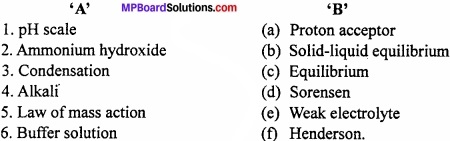1. (d)
2. (e)
3. (b)
4. (a)
5. (c)
6. (f)

[II]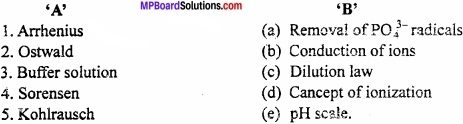1. (d)
2. (c)
3. (a)
4. (e)
5. (b)

Equilibrium Very Short Answer Type Questions

Question 1.
For unit of Kc the concentration is expressed as?
mol/litre.

Question 2.
Ammonia when dissolve in water give NH4OH, here the water acts as?
Like acid.

Question 3.
When NH4Cl is mixed with NH4OH solution, then the ionisation of NH4OH decreases. What is the reason?
Due to Common ion effect.

Question 4.
The pH of water at 25°C pH = 7. If water is heated upto 50°C, then what will be the change in pH?
The value of pH decreases.Question 5.
Write the conjugate base of H2PO4 and HC03?
HPO42- and CO32-.

Question 6.
Write the name of an ion which acts as both Bronsted acid and base?
HCO3.

Question 7.
Give an example of a salt formed by weak acid and weak base?
Ammonium acetate.

Question 8.
What is the pH value of human blood?
7.4.

Question 9.
What will happen when HCl gas is passed through NaCl?
NaCl will precipitate.

Question 10.
Among conjugate bases CN and F, which is stronger base?
CN is stronger base.Question 11.
What is the change in free energy (∆G) of reversible process at equilibrium?
Zero (0).

Question 12.
What is solubility product?
The product of the concentration of ions in the saturated solution of a sparingly soluble salt is a constant at a given temperature and is called solubility’ product.

Question 13.
What is buffer solution?
The solution in which on adding acid or base in iess quantity, the pH change is negligible, is called buffer solution.

Question 14.
In the exothermic reaction of ammonia formation N2(g) + 3H2(g) ⇄ 2NH3(g), when be the formation of ammonia will be more?
At low temperature and high pressure the formation of anunorua is more.

Question 15.
Give an example of acidic buffer?
Mixture of acetic acid and sodium acetate.Question 16.
What is the condition for precipitation?
For this ionic product should he more than solubility product.

Question 17.
What is the nature of aqueous solution of KCN?
It is of basic nature.

Question 18.
What is the nature of aqueous solution of CH3COONH4?
It is of neutral nature.

Question 19.
What will be the relation between Kc and Kp for the reaction
PCl5 ⇄ PCl3 + Cl2
Kp > Kc.

Question 20.
What is Lewis concept?
Acid is an electron pair acceptor and base is an electron pair donor.Question 21.
What is the definition of acid and base according to Bronsted and Lowry concept?
Acid is that which furnishes proton and base accepts the proton.

Question 22.
What is pH?
The pH value of a solution is the numerical value of the negative power to which 10 should be raised in order to express the Hydrogen ion concentration of the solution i.e. [H+] = 10-pH or pH = – log [H+].

Question 23.
The Ostwald dilution law applied on which electrolyte?
On weak electrolytes.

Equilibrium Short Answer Type Questions – I

Question 1.
Explain the effects of catalyst at equilibrium?
Effect of catalyst:
Le – Chatelier’s principle ignores the presence of a catalyst since the catalyst cannot displaces the equilibrium and simply reduce the time required for attaining the equilibrium when a catalyst is added to a reversible reaction in equilibrium. (MPBoardSolutions.com) It increases the speed of both forward and backward reaction i.e. rf and rb to the same extent. However the addition of a catalyst reduces the time required for a reaction to attain the equilibrium.

Question 2.
Explain Ostwald’s law of dilution?
Ostwald’s time dilution law:
Ostwald gave law for weak elecrolytes. By applying law of mass action Ostwald gave a law for expressing the dissociation of weak electrolyte. It states that:
“The degree of dissociation of weak electrolyte is directly proportional to the square root of its dilution.”
α = $$\sqrt { KV }$$ = $$\sqrt { \frac { K }{ C } }$$
Where, α = Degree of dissociation, K = Dissociation constant, V = Volume in litre in which one gram equivalent is dissolved, C = No. of gram equivalent in one litre.

Question 3.
What is the effect of pressure on chemical equilibrium?
On increasing the pressure on chemical equilibrium, the equilibrium shifts in that direction where the volume decreases i.e., the number of moles decreases.
Example: On combining of SO2 and O2, SO3 is formed and 45.2 kcal energy is liberated.
2SO2(g) + O2(g) ⇄ 2SO3(g); ∆H = -45.2 kcal
In this reaction, 2 moles of SO2 react with 1 mole of O2 to form 2 moles of SO3. So, on increasing the pressure the reaction shifts toward forward direction.Question 4.
What do you mean by buffer solution?
Buffer solution: Solution in which,

1. pH value is definite.
2. pH is not changed on dilution or on keeping for sometime.
3. On adding acid or base in less quantity, pH change is negligible.

Such solutions are called buffer solutions.
Or
Buffer solutions are solutions which retain their pH constant or unaltered.

Question 5.
What are acidic buffer and basic buffer?
1. Acidic buffers:
Acidic buffers are formed by mixing an equimolar quantities of weak acid and its salt with a strong base.
Example: Acetic acid and sodium acetate, boric acid and borax, citric acid and so-dium citrate, etc.

2. Basic buffers:
Basic buffers contain equimolar quantities of weak base and its salt with strong acid.
Example: Ammonium hydroxide and ammonium chloride.

Question 6.
On the basis ofthe equation pH = – log[H+], the pH of the 10-8mol dm-3 HCl solution should be pH = 8. But the observed value is less than 7. Explain the reason?
The 10-8moldm-3HCl shows that the solution is very dilute. So, we cannot neglect the H3O+ ions formed from water in the solution. So, the total [H3O+ ] = (10 -8 + 10-7) M comes to be near 7 or less than 7 i.e., the pH is less than 7. (As the solution is acidic).

Question 7.
Ammonia is a Lewis base. Why?
According to Lewis base, a base, is a substance (molecule or ion) which can donate an electron pair to form a co – ordinate. In the structure of ammonia, nitrogen is present in one lone pair of electron. So, ammonia donate a lone pair electron.
For example: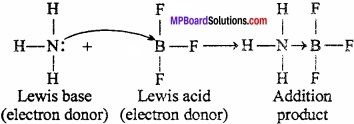Question 8.
Write the uses of Buffer solution?
Uses of Buffer solution:

1. To keep the pH of the reaction constant during the determination of velocity of chemical reactions.
2. The pH should be between pH – 5 to 6 – 8 in the formation of alcohol by fermentation.
3. Preparation of sugar, paper and electroplating occurs at definite pH.Question 9.
What is the effect of pressure and temperature on solubility of gases in liquids?
1. Effect of Pressure:
On increasing the pressure the solubility of gases increases as the molecules of gases enters in the intermoiecular spaces of solvent.

2. Effect of Temperature:
On increasing the temperature the solubility of gases decreases as the kinetic energy of gas molecules increases.

Question 10.
Write the name of the factors affecting the chemical equilibrium?
The factors affecting the chemical equilibrium are:

1. Temperature
2. Pressure
3. Change in concentration
4. Catalyst.

Question 11.
Why the solubility of C02 decreases on Increasing the temperature?
CO2(g) + aq ⇄ CO2(eq)
The solubility of CO2 in water is an exothermic reaction. So, according to Le – Chatelier’s principle, on increasing temperature the reaction proceeds towards backward direction. That is why on increasing temperature the solubility of CO2 decreases.

Question 12.
What do you understand by ionization of water?
Auto ionisation occurs in water molecules. The ionic equilibrium of water can be shown by following equation:
H2O + H2O ⇄ H3OH+ + OH
Equilibrium constant K = $$\frac { [H_{ 3 }O^{ + }][OH^{ – }] }{ [H_{ 2 }O]^{ 2 } }$$
⇒ KH2O = [H3O+][OH] [∵ KH2O = Kw]
Kw = 1 × 10-14
Here Kw is a constant, called ionic product of water.

Question 13.
What is concentration Quotient?
The ratio of concentration of products and reactants is called concentration quotient. It is denoted by Q. For any reversible reaction the concentration quotient is equal to the equilibrium constant Kc.
Kc = $$\frac { [C]^{ c }[D]^{ d } }{ [A]^{ d }[B]^{ b } }$$
and Q = $$\frac { [C_{ C }]^{ c }[C_{ D }]^{ d } }{ [C_{ A }]^{ d }[C_{ B }]^{ b } }$$
At equilibrium Q = Kc.Question 14.
What do you understand by Lewis acid and Lewis base? Explain with example?
Lewis acid:
Acid is a substance (an atom, molecule or ion) which can accept a pair of electrons to complete its octet.
Example: BF3, AlCl3, Br+, NO2+ etc.

Lewis base:
Lewis base is a substance (an atom, molecule or ion) which have complete octet of the central metal atom and have a lone pair of electron to donate in a chemical reaction to form co – ordinate bond.
Example:Question 15.
At 310 K, ionic product of water is 2.7 × 10-14. at this temperature determine the pH of neutral water?
Solution:
Kw = [H3O+].[OH] = 2.7 × 10-14 (At 310 K)
For reaction H2O + H2O ⇄ [H3O+] [OH]
[H3O+] = [OH]
So, [H3O+] = $$\sqrt { 2.7\times 10^{ -14 } }$$ = 1.643 × 10-7M
pH = -log [H3O+] = -log 1.643 × 10-7
= 7 + (- 0.2156) = 6.7844.

Question 16.
Determine the concentration quotient for following reactions:

1. CrO4-2(eq) + Pb(eq) ⇄ PbCrO4(s)
2. CaC03(s) ⇄ CaO(s) + CO2(g)
3. NH3(eq) + H2O(l) ⇄ NH4+(eq) + OH(eq)
4. H2O(l) ⇄ H2O(g)Question 17.
What is the importance of solubility product in precipitation of soap?
Soap is produced on alkaline hydrolysis of oil and fats. Soaps are actually sodium or potassium salts of higher fatty acids. (MPBoardSolutions.com) Soap is obtained in the form of concentrated liquid. Concentrated salt solution is added for its precipitation. In presence of increased concentration of common sodium ion, ionic product of [Na+] [Cn H2n+1 COO] exceeds its solubility product (Ksp) and it gets precipitated. It is separated by filtration.

Equilibrium Short Answer Type Questions – II

Question 1.
By comparing the values of Kc and how will you find out the following state of the reaction:

1. Resultant reaction proceeds towards forward direction.
2. Resultant reaction proceeds towards backward direction.
3. No change in the reaction.

1. If Qc < Kc; reaction proceeds towards the direction by products (Forward reaction).
2. If Qc > Kc; reaction proceeds towards reactant side (Backward reaction).
3. If Qc = Kc; at equilibrium, the reaction mixture remains as before. So no change in the reaction.Question 2.
The aqueous solution of sodium carbonate is basic in nature, why?
Na2CO3 ⇄ 2Na+ + CO3-2
2H – OH ⇄ = 2H+ + 2OH
Na2CO3 + 2H – OH ⇄ 2NaOH + H2CO3
Neither solid Na2CO3 nor water alone has any action on litmus paper. However aqueous solution of Na2CO3 turned red litmus blue. The problem is successfully explained by the Arrhenius theory of ionization in terms of hydrolysis, when a salt is dissolved in water, it undergoes ionization. (MPBoardSolutions.com) The ions of salt interact with opposite ion of water to form acidic basic or neutral solution. This process is called hydrolysis may be defined as the interaction of ion of a salt with oppositely charged ion of water to give acidic or basic solution. Consider the hydrolysis of a general salt (AB).
Dissociation of salt
AB +Water → A+ + B
Dissociation of water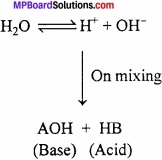Question 3.
What is the effect on equilibrium when gases are dissolved in liquids? Explain with example?
When the soda water bottle is opened, dissolved CO2 gas in it comes out rapidly. This is an example of equilibrium of any gas in equilibrium between gas and its liquid. At finite pressure of the gas there exists an equilibrium between soluble and insoluble molecules of the gas.
CO2(g) ⇄ CO2(eq)
Henry’s law: The solubility of gas in a given solvent is directly proportional to the pressure to which the gas is subjected, provided the temperature remains the same.
Thus, m ∝ P
or m = KP
Where, K is a constant of proportionality and known as Henry’s constant.
Example: You have seen that when a soda water bottle is opened, the carbon dioxide dissolved in it freezes out rapidly. This can be explained on the basis of Henry’s law. (MPBoardSolutions.com) Carbonated beverages are bottled under pressure to ensure high concentration of carbon dioxide. When the bottle is opened the pressure above the solution falls and the excess carbon dioxide comes out.

Question 4.
What is common ion effect? Explain?
Common ion effect:
When, in a solution of weak electrolyte, a solution of strong electrolyte containing the same ion is added, then the ionisation of weak electrolyte decreases. This effect is called common ion effect.
Example: During precipitation of radicals of group II taken care that the radicals of group IV does not get precipitated. (MPBoardSolutions.com) On the basis of this, H2S gas is passed in presence of HCl in group II. Due to common ion effect dissociation of H2S is suppressed.This reduces concentration of sulphide ion and hence only radicals of group II get precipitated as sulphide. For the precipitation of radicals of group IV, H2S is passed in presence of NH4OH. H+ ion of H2S combines with OH ions of NH4OH to produce water. This increases dissociation of H2S. In this way, increase in sulphide ion concentration helps in the precipitation of positive sulphides of group IV.Question 5.
What do you understand by conjugate acid and conjugate base?
The relative strengths of conjugate pairs can be found out, if we know whether forward reaction is favoured or backward reaction is favoured.
For example:In the above reaction, the reaction proceeds almost to completion. We must therefore conclude that HCl is a stronger acid than H3O+ i.e., HCl has stronger tendency to donate proton than H3O+. Similarly H2O is stronger base than H2O has stronger tendency to accept proton than Cl. Thus, the strong acid (HCl) has a weak conjugate base Cl.
Consider another reactionFor the reaction, backward reaction is strongly favoured. This shows that H3O+ is a stronger acid than CH3COOH and CH3COO is a stronger base than H2O. Thus, we again observe that the strong acid (H3O+) has the weak conjugate base (H2O). Thus, we conclude that, A stronger acid has a weak conjugate base and vice – versa.

Question 6.
Explain the solubility product by giving definition?
Solubility product:
The product of the concentration of ions in the saturated solution of a sparingly soluble salt as AgCl is a constant at a given temperature and is called solubility product. (MPBoardSolutions.com) If any sparingly soluble electrolyte at any temperature forms saturated solution, then the equilibrium isAccording to law of mass action from (b) and (c),
$$\frac { [A^{ + }][B^{ + }] }{ [AB] }$$ = K
∴ [A+ ][B+ ] = K[AB]
When solution is saturated [AB] will be constant and value of K[AB] will also be a constant and is written as KJ which is the solubility product.

Question 7.
Explain the acid – base concept of Bronsted – Lowry by giving example?
Bronsted – Lowry concept of acid and base (1923):
Scientist Bronsted and Lowry gave the theory for acids and bases which is equally applied to aqueous and non – aqueous solutions of acids and bases. According to it, “Acid is that which furnishes proton and base accepts the proton.”

Neutralization reaction:
A reaction in which a proton is transferred from acid to a base or a reaction between H+ and OH ion to form H2O molecule.
Relation between acid and base:That means, every acid has conjugate base and every base has conjugate acid.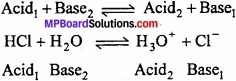According to the definition given by Bronsted and Lowry, acid and base may be in molecular, cationic or anionic states.

Question 8.
Calculate the value of equilibrium constant for following reaction:
PCl5 ⇄ PCl3 + Cl2
Let ‘a’ mole of PCl5, initiate the reaction and x moles dissociate at equilibrium state. If the volume of container is V litre then,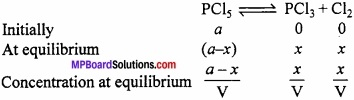According to law of mass of action
Kc = $$\frac { [PCl_{ 3 }][Cl_{ 2 }] }{ [PCl_{ 5 }] }$$
On putting the values,Question 9.
With the help of Le – Chatelier’s principle at equilibrium for reaction 2SO3 + O2 ⇄ 2SO3;
∆H = – 188.2 kJ, determine the restrictions for the formation of sulphur trioxide?
Le – Chatelier proposed a law. It states “If as equilibrium m £ chemical system is disturbed by changing temperature, pressure or concentration of the system, the equilibrium shift is such a way so that the effect of change gets minimised.”
On the basis of this principle, changes in chemical equilibria and physical equilibria can be explained.
Formation of SO3 by SO2 and O2:
2SO2 + O2 ⇄ 2SO3; ∆H = – 45.2 kcal

1. Effect of concentration:
On increasing concentration of SO2 and O2 more SO3 will be formed.

2. Effect of pressure:
On increasing pressure, the equilibrium shifts on that side in which number of moles are less or towards less volume. In this reaction, 2 moles of SO2 and 1 mole of O2 (Total 3 moies) change into 2 moles of SO3, So, on increasing pressure, more quantity of SO3 will be formed.

3. Effect of temperature:
Formation of SO3 is an exothermic reaction, so on increasing temperature, more SO3 will dissociate and on decreasing temperature, more SO3 will be formed.

Question 10.
What is the importance of solubility product m the purifkation of salt?
Purification of common salt; Common salt contains impurities in it. For removal of these HCl gas is passed through the saturated solution of salt. HCl ionizes tc greater extent being a strong electrolyte.
HCl ⇄ H+ + Cl (More ionized)
NaCl ⇄ NaCl ⇄ Na+ + Cl

Equilibrium Long Answer Type Questions – I

Question 1.
Explain the buffering action of alkaline or basic buffer? Give its importance?
Basic buffer contains equimolar quantities of weak base and sodium citrate etc.
Buffer action of basic buffer: Consider a basic buffer of NH4OH and NH4Cl. This buffer solution contains a large amount of NH4+ ions, Cl ions and excess of undissociated NH4OH molecules along with a small amount of OH ions.
NH4Cl → NH4+ + Cl
NH4OH ⇄ NH4+ + OH
On adding a drop of HCl, H3O+ ions produced combines with OH ions of the buffer to form weakly ionised H2O molecules. As a result of this ionisation of NH4OH increases to restore the concentration of OH ions. Sc, pH of solution remains unchanged.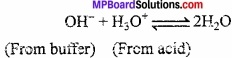When a few drops of NaOH is added, it provides OH ions. The additional OH ions combines with NH4+ ions to form weakly ionised NH4OH resulting an increase in ionisation of NH4OH to restore the concentration of NH4+ ion. Thus, pH remains unchanged.Importance of Buffer solution:

1. Buffer solutions are very important in laboratories, industries, botanical or zoological, physiology. Human blood is an example of buffer solution which has pH 7.34.
2. In the extraction of phosphate the buffer solution of CH3COONa and CH3COOH is used.
3. In industries, for the manufacturing of sugar and paper and electroplating occurs at fixed pH.Question 2.
What is pH? Explain?
Or, What is meant by pH? What is its relationship with hydrogen ion concentration?
To express the acidity or basicity of a solution Sorensen in 1909 established a scale known as the pH scale.
The pH value of a solution is the numerical value of the negative power to which 10 should be raised in order to express the hydrogen ion concentration of the solution, i.e;
[H+] = 10-pH …………… (1)
On taking log of eqn.(1),
or log10[H+] = log10 10-pH
log10[H+] = -pH log10 10
or pH = -log [H+], (∵ log10 10 = 1)
or pH = log10$$\frac { 1 }{ [H^{ + }] }$$
This is the required relationship between pH and H+ ion concentration.
Thus, the pH value of a solution is the logarithm of its hydrogen ion concentration to base 10 with negative sign.
pH scale expresses acidic and basic nature of solution in terms of numbers from 0 to 14. Acidic solutions have pH value less than 7 while basic solutions have pH value greater than 7. pH value of neutral solution is 7.
Uses of pH measurement:

1. In industries:
• In the manufacturing of alcohol by fermentation, pH is maintained between 5 and 6.8.
• Manufacturing of paper, sugar and electroplating is being done at fixed pH.
2. In the study of velocities of chemical reactions, for maintaining pH, buffer solutions are used.
3. In qualitative analysis for removal of phosphate to maintain pH, buffer solutions of CH3COONa and CH3COOH are used.
4. pH of human blood is 7.34. Medium of stomach is acidic while in the intestine it is basic medium.

Question 3.
Write the characteristics of equilibrium constant?
Characteristics of equilibrium constant:
1. The value of equilibrium constant for a reaction is constant at a given temperature and it changes with change in temperature.

2. The value of molar equilibrium constant does not depends upon the initial molar concentration of reactants and products but it depends upon their concentration at equilibrium state.

3. If the reaction is reversed the value of equilibrium constant is inversed. For example,
Then for, H2(g) + I2(g) ⇄ 2HI, Kc = 50
2HI(g) ⇄ H2(g) + I2(g)
∴ K’ = $$\frac { 1 }{ K }$$ or K’c = $$\frac{1}{50}$$ = 0.02

4. If the equation having equilibrium constant is divided by 2, the equilibrium constant for the new equation is the square root of K (i.e., $$\sqrt { K }$$)
For example:5. If the equation (Having equilibrium constant K) is multiplied by 2, the equilibrium constant for the new equation is square of K (i. e., K2)
K’ = K2

Question 4.
Give only the definition of:

1. Salt hydrolysis
2. Solubility product
3. Common ion effect
4. Buffer solution

1. Salt hydrolysis:
The interaction of cation/anion or both with water making the solution acidic or basic is called salt hydrolysis.

2. Solubility product:
At a particular temperature the product of concentration of ions in a saturated solution of a sparingly soluble electrolyte is known as solubility product. (Ksp).

3. Common ion effect:
The dissociation of weak electrolyte (Weak acid or weak base) is suppressed by the addition of a strong electrolyte having a common ion is called common ion effect.

4. Buffer solution:
Buffer solutions are solutions which retain their pH constant or unaltered after addition of less quantity of acid or base.Question 5.
Before precipitation of IIIrd group radicals as hydroxides on adding NH4OH, why it is necessary to add NH4Cl first?
Precipitation of hydroxide of group ill:
Radicals of group III i e.. Al3+, Fe3+, Cr3+ gets precipitated as hydroxides. Radicals cf succeeding groups such as Zn2+, Ni2+, Mg2+, etc. are also precipitated as hydroxide. (MPBoardSolutions.com) Solubility product of hydroxide of group III is lesser than that of other as given below:
Table: Solubility Product of Hydroxide at 18°CDue to the difference in the solubility product of the hydroxides, concentration of OH ion is kept low such that only radicals of group III gets precipitated.
Hence, NH4OH is added before adding NH4Cl which remains completely ionized. Due to common (NH4+) ion dissociation of weak electrolyte NH4OH is suppressed.
NH4Cl ⇄ NH4+ + Cl (More ionised)
NH4OH ⇄ NH4+ + OH (Less ionised)
This decreases comcentration of OH ion and only hydroxides with low solubility product gets precipated.

Equilibrium Long Answer Type Questions – II

Question 1.
Derive a relation between equilibrium constant Kp and Kc, Or, Prove that Kp = KcRT∆n?
In year 1867 Guldberg and Waage put forward a relationship between the rate of reaction and the molar concentration of reactants. The reUucrjhip is.0 as law of mass action. (MPBoardSolutions.com) It states that “At constant temperature, the rate of a chemical reaction is directly proportional to the product of the molar concentration of the reaction each raised to a power equal to its corresponding stoichiometric coefficient that appears in the balanced chemical equation.”
Example: For solids and liquids: Consider a hypothetical reversible reaction in the state of equilibrium.
A + B ⇄ X + Y
Applying law of mass action: Rate of forward reaction
Rf ∝ [A] [B] or Rf = Kf[A][B] ……………… (1)
Where, Kf is rate constant or velocity constant for the forward reaction. Similarly, rate of backward reaction,
Rb ∝ [X] [Y] or Rb = Kb [X][Y] ………………… (2)
Where, Kb rate constant or velocity constant for the backward reaction.
At equlibrium,
Rate of forward reaction = Rate of backward reaction.
Kf[A][B] = Kb[X][Y]Its value remains constant at particular temperature. The equation (3) is called law of chemical equilibrium. For a general type of the reaction,
aA + bB ⇄ xX + yYFor gaseous reactants and products:
In the expression for Kc, the concentrations of the various species are generally expressed in terms of moles/litre. (MPBoardSolutions.com) However, in case of gaseous reactions the concentrations of gases may also expressed in terms of their partial pressures. Therefore, for a gaseous reaction:
aA + bB ⇄ xX + yY
The law of chemical equilibrium may be expressed as:Relationship between Kpc:
Let us consider the following general reaction,
aA + bB ⇄ xX + yY
In which all the substances A, B, X and Y are present in gaseous state. For this reaction, Kp and Kc is written as follows:Since, for an ideal gas,
PV = nRT
∴ P = $$\frac{n}{V}$$ RT = CRT ………………… (3)
Where, the term C (equal to $$\frac{n}{V}$$) represents the molar concentration of the gas. On the basis of equation (3), we have
PA = CART = [A] RT
PB = CBRT = [B] RT
PX = CX RT = [X] RT
PY = CY RT = [Y] RT
Substituting this value in equation (2), we get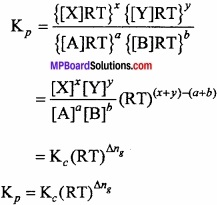Proved.Question 2.
Derive Ostwald’s dilution law of ionization of weak electrolytes? Write its limitations?
Or, Deduce the relationship between degree of ionization and ionization constant?
Ostwald’s dilution law: Weak electrolytes are partially ionised. The ions produced due to ionisation of weak electrolyte exist in dynamic equilibrium with the undissociated molecules.
The fraction of the total number of molecules of electrolyte dissolved, which ionises at equilibrium is called degree of ionisation or degree of dissociation. It is denoted by α.
Consider ‘C’ mol per litre be the initial concentration of weak electrolyte AB dissolved in water. Let α be its degree of ionisation. (MPBoardSolutions.com) Thus, the molar concentration of different species before ionisation and at equilibrium be as given below: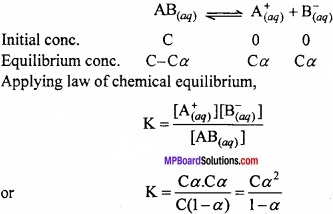Under normal conditions, value of a is very small for weak electrolyte and it can be neglected in comparison to 1.
∴K = Cα2
or α2 = $$\frac{K}{C}$$
∴α = $$\sqrt { \frac { K }{ C } }$$
If V is the volume of the solution in litres containing 1 mole of electolyte, then C = $$\frac{1}{V}$$. Thus,
α = $$\sqrt { KV }$$
The above equation helps us to conclude that:
The degree of ionisation is inversely proportional to the square root of the molar concentration or directly propotional to the square root of the volume of the solution containing one mole of electrolyte. This is called Ostwald’s dilution law.

Limitations:
This law is not applicable for strong electrolyte and concentrated solutions because attractive force also acts between the solutions of such electrolytes.

Question 3.
Derive a relationship between pH and pOH value? Or, Prove that pH + pOH = 14.
The water ionizes as:
H2O + H2O ⇄ H3O+ + OH
K = $$\frac { [H_{ 3 }O^{ + }][OH^{ – }] }{ [H_{ 2 }O]^{ 2 } }$$
K [H2O ]2 = [H3O+] [OH]
[∵K [H2O ]2 = Kw]
Kw = [H3O+][OH] ……………. (1)
Kw is a constant, it is called product solubility.
At 298K, Temperature Kw = 1 × 10-14.
On putting the value in equation (1)
10-14 = [H3O+][OH]
Taking log on both sides,
-14 log1010 = log10[H3O+] + log10[OH]
-14 = log10[H3O+] + log10[OH], [∵log1010 = 1]
0r 14 = [-log10[H3O+]] + [-log10[OH]]
[∵log10 [H3O+] = pH]
[∵log10 [OH] = pOH]
14 = pH + pOHQuestion 4.
For the determination of pH value of buffer solution derive Henderson – Hazel equation?
Henderson’s equation:
pH of a buffer solution can be calculated with the help of Henderson’s equation. For this consider a buffer of weak acid HA and its salt.
HA ⇄ H+ + A
Ka = $$\frac { [H^{ + }][A^{ – }] }{ [HA] }$$
Ka is dissociation constant of acid.
or [H+] = Ka $$\frac { [HA] }{ [A^{ – }] }$$
Salt is completely ionised while due to presence of excess A from the salt, the dissociation of weak acid will be depressed more due to common ion effect.
or [H+] = Ka $$\frac { [Acid] }{ [Salt] }$$ [A] ≈ [Salt]
Taking log value,This equation is called Henderson equation.
In the same way, for basic buffer solution:pH of a buffer solution does not change woth dilution because on dilution the ration of conc. of acid or base does not change.